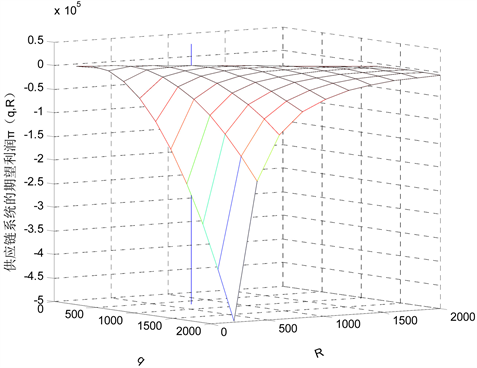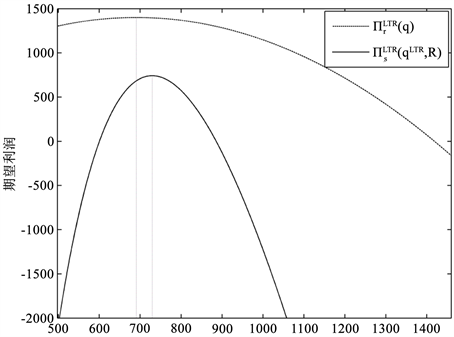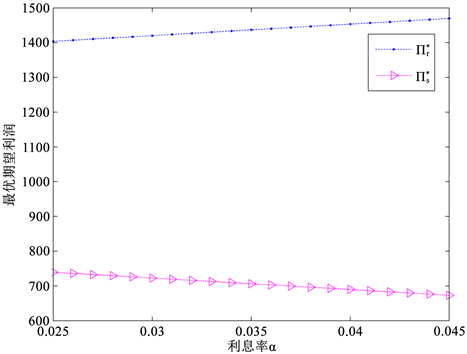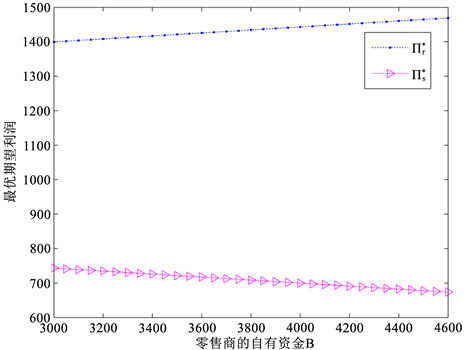﻿ 供需不确定环境下零售商订购资金有限的供应链协调 Supply Chain Coordination with Limited Order Funds for Retailer under Supply and Demand Uncertainty

Operations Research and Fuzziology
Vol. 09  No. 01 ( 2019 ), Article ID: 28509 , 14 pages
10.12677/ORF.2019.91003

Supply Chain Coordination with Limited Order Funds for Retailer under Supply and Demand Uncertainty

Ping Sun, Jiangtao Mo*, Yujin Zhong

College of Mathematics and Information Science, Guangxi University, Nanning GuangxiReceived: Dec. 26th, 2018; accepted: Jan. 10th, 2019; published: Jan. 17th, 2019ABSTRACT

This paper studies the coordination of a supply chain with supply and demand uncertainty. The supply chain is consisting of a supplier and, a retailer who has limited order funds. The research shows that, under the condition of credit payment, the retailer can break through the restriction of funds and increase his order quantity, then supply chain profit is improved. But the supply chain cannot be coordinated. Applying credit payment with revenue sharing contract together, even if the retailer uses his limited order funds only, the coordination of the supply chain can be achieved. Moreover, compared with the case of supply certainty and demand uncertainty, the wholesale price is higher or the profit sharing ratio is lower during coordination. Finally, the theoretical results are verified by numerical examples, and the influences of supply and demand fluctuation on supply chain decision and profit are also analyzed.

Keywords:Supply and Demand Uncertainty, Limited Order Funds, Credit Payment, Revenue Sharing Contract, Supply Chain Coordination1. 引言

2. 问题与基本假设

1) 供应商提供产品，零售商订购产品并销售给顾客；供应商和零售商是风险中性和信息共享的，决策目标是最大化各自的期望利润。

2) 供应商的资金是无限的，零售商的订购资金是有限的，即零售商用于订购产品的资金是有限的，记为 $B$

3) 市场对产品的需求 $x$是随机的，其密度函数和分布函数分别为 $f\left(x\right)$$F\left(x\right)$

4) 产品的供应率 $\epsilon$是随机的， $\epsilon \in \left[a,b\right]$，且 $0，其密度函数和分布函数分别为 $g\left(\epsilon \right)$$G\left(\epsilon \right)$，均值为 ${\mu }_{\epsilon }$

5) 零售商的订购量为 $q$，单位产品的销售价格为 $p$

6) 供应商的单位生产成本为 $c$，单位批发价为 $w$；供应商的计划生产量为 $R$，但实际生产量是 $\epsilon R$。当 $\epsilon R时，供应商从现货市场采购 $q-\epsilon R$单位产品，单位采购成本为 $e$

7) 忽略未售出单位产品的残值和缺货成本，假设 $p>w>c>0$$e{\mu }_{\epsilon }>c$

8) 供应商提供信用支付鼓励零售商订购更多产品，即零售商在订购产品时，先支付订购资金 $B$，剩余部分在销售期末支付，并且要支付利息，利息率为 $\alpha$。为使零售商接受该契约，从中盈利，需假设 $p>\left(1+\alpha \right)w$

9) 在供应商提供信用支付的情况下，在销售期末，可能出现零售商的销售收入不足以支付剩余货款及利息的情况，此时，零售商需要利用其他资金偿还剩余货款及利息。

1) 零售商和供应链系统的期望利润同时达到最优；

2) 零售商和供应商的期望利润都不低于各自的保留利润；

3. 集中式供应链

$\begin{array}{c}E{\text{π}}_{c}\left(q,R\right)=pE\mathrm{min}\left(q,x\right)-eE{\left(q-\epsilon R\right)}^{+}-cR\\ =pq-p{\int }_{0}^{q}F\left(x\right)\text{d}x-eR{\int }_{a}^{q/R}G\left(\epsilon \right)\text{\hspace{0.17em}}\text{d}\epsilon -cR.\end{array}$(1)

${q}^{C}={F}^{-1}\left(\frac{p-{c}_{z}}{p}\right)$${R}^{C}=\frac{1}{z}{q}^{C}$(2)

${\int }_{a}^{z}\epsilon g\left(\epsilon \right)\text{\hspace{0.17em}}\text{d}\epsilon =\frac{c}{e}$(3)

$E{\text{π}}_{c}\left({q}^{C},R{}^{C}\right)=\left(p-{c}_{z}\right){q}^{C}-p{\int }_{0}^{{q}^{C}}F\left(x\right)\text{d}x$(4)

4. 分散式供应链

4.1. 批发价契约下无信用支付的情形

$\begin{array}{c}E{\text{π}}_{r}\left(q\right)=pE\mathrm{min}\left(q,x\right)-wq\\ =\left(p-w\right)q-p{\int }_{0}^{q}F\left(x\right)\text{d}x.\end{array}$(5)

$\begin{array}{ll}\mathrm{max}\hfill & E{\text{π}}_{r}^{}\left(q\right)\hfill \\ s.t.\hfill & 0\le q\le {q}^{W}\hfill \end{array}$

$\begin{array}{c}E{\text{π}}_{s}\left(q,R\right)=wq-eE{\left(q-\epsilon R\right)}^{+}-cR\\ =wq-eR{\int }_{a}^{q/R}G\left(\epsilon \right)\text{\hspace{0.17em}}\text{d}\epsilon -cR.\end{array}$(6)

${q}^{I}={F}^{-1}\left(\frac{p-w}{p}\right)$${R}^{I}={q}^{I}/z$(7)

2) 记使订购资金有限的零售商、资金无限的供应商期望利润最优的订购量和计划生产量分别为 ${q}^{L}$${R}^{L}$，则

${q}^{L}=\mathrm{min}\left\{{q}^{I},{q}^{W}\right\}$${R}^{L}={q}^{L}/z$(8)

$E{\text{π}}_{s}\left({q}^{L},{R}^{L}\right)=\left(w-{c}_{z}\right){q}^{L}$(9)

${q}^{L}\le {q}^{I}={F}^{-1}\left(\frac{p-w}{p}\right)<{F}^{-1}\left(\frac{p-{c}_{z}}{p}\right)={q}^{C}$

${R}^{L}={q}^{L}/z<{q}^{C}/z={R}^{C}$

4.2. 批发价契约下有信用支付的情形

$\begin{array}{c}E{\text{π}}_{r}^{LT}\left(q\right)=pE\mathrm{min}\left(q,x\right)-\left(wq-B\right)\left(1+\alpha \right)-B\\ =\left(p-\left(1+\alpha \right)w\right)q-p{\int }_{0}^{q}F\left(x\right)\text{\hspace{0.17em}}\text{d}x+\alpha B.\end{array}$(10)

$\begin{array}{c}E{\text{π}}_{s}^{LT}\left(q,R\right)=B+\left(wq-B\right)\left(1+\alpha \right)-eE{\left(q-\epsilon R\right)}^{+}-cR\\ =\left(1+\alpha \right)wq-eR{\int }_{a}^{q/R}G\left(\epsilon \right)\text{\hspace{0.17em}}\text{d}\epsilon -cR-\alpha B.\end{array}$(11)

$\begin{array}{ll}\mathrm{max}\hfill & E{\text{π}}_{r}^{LT}\left(q\right)\hfill \\ s.t.\hfill & {q}^{W}\le q\hfill \end{array}$

${q}^{LT}\text{=}\mathrm{max}\left({q}^{Lt},{q}^{W}\right)$${R}^{LT}={q}^{LT}/z$(12)

${q}^{Lt}={F}^{-1}\left(\frac{p-\left(1+\alpha \right)w}{p}\right)$(13)

$\frac{\text{d}E{\text{π}}_{r}^{LT}\left(q\right)}{\text{d}q}=p\left[\text{1}-F\left(q\right)\right]-\left(1+\alpha \right)w$(14)

$\frac{{\text{d}}^{2}E{\text{π}}_{r}^{LT}\left(q\right)}{\text{d}{q}^{2}}=-pf\left(q\right)$(15)

$q\to 0$时， $\frac{\text{d}E{\text{π}}_{r}^{LT}\left(q\right)}{\text{d}q}\to p-\left(1+\alpha \right)w$；当 $q\to \infty$时， $\frac{\text{d}E{\text{π}}_{r}^{LT}\left(q\right)}{\text{d}q}\to -\left(1+\alpha \right)w$。由 $p>\left(1+\alpha \right)w$可得， $p-\left(1+\alpha \right)w>0$$-\left(1+\alpha \right)w<0$，则存在唯一 ${q}^{Lt}\in \left(0,\infty \right)$满足(13)式，使 $\text{d}E{\text{π}}_{r}^{LT}\left(q\right)/dq=0$。再由 $f\left(x\right)>0$可得， ${\text{d}}^{2}E{\text{π}}_{r}^{LT}\left(q\right)/\text{d}{q}^{2}<0$，即 $E{\text{π}}_{r}^{LT}\left(q\right)$是关于 $q$的凹函数。又因 $q\ge {q}^{W}$，因此， ${q}^{LT}=\mathrm{max}\left({q}^{Lt},{q}^{W}\right)$

$E{\text{π}}_{s}^{LTR}\left(q,R\right)$求关于 $R$的一阶和二阶导数可得：

$\frac{\text{d}E{\text{π}}_{s}^{LT}\left(q,R\right)}{\text{d}R}=e{\int }_{a}^{q/R}\epsilon g\left(\epsilon \right)\text{\hspace{0.17em}}\text{d}\epsilon -c$(16)

$\frac{{\text{d}}^{2}E{\text{π}}_{s}^{LT}\left(q,R\right)}{\text{d}{R}^{2}}=-\frac{e{q}^{2}}{{R}^{3}}g\left(\frac{q}{R}\right)$(17)

$g\left(\epsilon \right)>0$可得， ${\text{d}}^{2}E{\text{π}}_{s}^{LT}\left(q,R\right)/\text{d}{R}^{2}<0$，即 $E{\text{π}}_{s}^{LT}\left(q,R\right)$是关于 $R$的凹函数。当 $R\to 0$时， $\frac{\text{d}E{\text{π}}_{s}^{LT}\left(q,R\right)}{\text{d}R}\to e{\mu }_{\epsilon }-c$；当 $R\to \infty$时， $\frac{\text{d}E{\text{π}}_{s}^{LT}\left(q,R\right)}{\text{d}R}\to -c$。由 $e{\mu }_{\epsilon }>c$可得， $e{\mu }_{\epsilon }-c>0$$-c<0$。因此，当 $q={q}^{LT}$时，存在唯一的 ${R}^{LT}={q}^{LT}/z$，使 $\text{d}E{\text{π}}_{s}^{LT}\left(q,R\right)/\text{d}R=0$，其中 $z$由(3)式定义。

$E{\text{π}}_{s}^{LT}\left({q}^{LT},{R}^{LT}\right)=\left(\left(1+\alpha \right)w-{c}_{z}\right){q}^{LT}-\alpha B$(18)

${q}^{Lt}={F}^{-1}\left(\frac{p-\left(1+\alpha \right)w}{p}\right)<{F}^{-1}\left(\frac{p-{c}_{z}}{p}\right)={q}^{C}$

${R}^{LT}={q}^{LT}/z<{q}^{C}/z={R}^{C}$

5. 信用支付和收益共享契约下的供应链

5.1. 供应链成员的最优生产订购决策

$\begin{array}{c}E{\text{π}}_{r}^{LTR}\left(q\right)=\phi pE\mathrm{min}\left(q,x\right)-\left(wq-B\right)\left(1+\alpha \right)-B\\ =\left(\phi p-\left(1+\alpha \right)w\right)q-\phi p{\int }_{0}^{q}F\left(x\right)\text{\hspace{0.17em}}\text{d}x+\alpha B.\end{array}$(19)

$\begin{array}{c}E{\text{π}}_{s}^{LTR}\left(q,R\right)=\left(1-\phi \right)pE\mathrm{min}\left(q,x\right)+B+\left(1+\alpha \right)\left(wq-B\right)-eE{\left(q-\epsilon R\right)}^{+}-cR\\ =\left(1+\alpha \right)wq\text{+}\left(1-\phi \right)p\left[q-{\int }_{0}^{q}F\left(x\right)\text{\hspace{0.17em}}\text{d}x\right]-eR{\int }_{a}^{q/R}G\left(\epsilon \right)\text{\hspace{0.17em}}\text{d}\epsilon -\alpha B-cR.\end{array}$(20)

${q}^{LTR}=\mathrm{max}\left({q}^{LTr},{q}^{W}\right)$${R}^{LTR}={q}^{LTR}/z$(21)

${q}^{LTr}={F}^{-1}\left(\frac{\phi p-\left(1+\alpha \right)w}{\phi p}\right)$(22)

$\frac{\text{d}E{\text{π}}_{r}^{LTR}\left(q\right)}{\text{d}q}=\phi p\left[1-F\left(q\right)\right]-\left(1+\alpha \right)w$(23)

$\frac{{\text{d}}^{2}E{\text{π}}_{r}^{LTR}\left(q\right)}{\text{d}{q}^{2}}=-\phi pf\left(q\right)$(24)

$\phi p>\left(1+\alpha \right)w$可得：

$\underset{q\to 0}{\mathrm{lim}}\frac{\text{d}E{\text{π}}_{r}^{LTR}\left(q\right)}{\text{d}q}=\phi p-\left(1+\alpha \right)w\ge 0$

$\underset{q\to \infty }{\mathrm{lim}}\frac{\text{d}E{\text{π}}_{r}^{LTR}\left(q\right)}{\text{d}q}=-\left(1+\alpha \right)w<0$

$E{\text{π}}_{s}^{LTR}\left({q}^{LTR},{R}^{LTR}\right)=\left[\left(1+\alpha \right)w-{c}_{z}\right]{q}^{LTR}+\left(1-\phi \right)p\left[{q}^{LTR}-{\int }_{0}^{{q}^{LTR}}F\left(x\right)\text{\hspace{0.17em}}\text{d}x\right]-\alpha B$(25)

5.2. 供应链协调

$\phi =\frac{1+\alpha }{{c}_{z}}w$(26)

$\phi p=\frac{p\left(1+\alpha \right)}{{c}_{z}}w>\left(1+\alpha \right)w$

${q}^{W}\le {q}^{C}$。于是， ${q}^{LTr}={q}^{C}\ge {q}^{W}$。因此， ${q}^{LTR}={q}^{LTr}={q}^{C}$。由定理4和定理1可知， ${R}^{LTR}={q}^{LTR}/z={q}^{C}/z={R}^{C}$，即定义1中的条件(1)得到满足。

$\left\{\begin{array}{l}E{\text{π}}_{r}^{*}\ge E{\text{π}}_{r}^{0};\\ E{\text{π}}_{s}^{*}\ge E{\text{π}}_{s}^{0}.\end{array}$(27)

$w=\frac{{c}_{z}}{1+\alpha }\phi$(28)

$\mathrm{max}\left({\phi }_{0},{\phi }_{1}\right)\le \phi \le {\phi }_{2}$(29)

${\phi }_{0}=\frac{\left(1+\alpha \right)B}{{c}_{z}{q}^{C}}$${\phi }_{1}=\frac{E{\text{π}}_{r}^{0}-\alpha B}{E{\text{π}}_{c}^{*}}$${\phi }_{2}=\frac{E{\text{π}}_{c}^{*}-E{\text{π}}_{s}^{0}-\alpha B}{E{\text{π}}_{c}^{*}}$

$E{\text{π}}_{r}^{*}=\phi E{\text{π}}_{c}^{*}+\alpha B$(30)

$E{\text{π}}_{s}^{*}=\left(1-\phi \right)E{\text{π}}_{c}^{*}-\alpha B$(31)

$\phi =\frac{1+\alpha }{c}w$(32)

${c}_{z}=eG\left(z\right)>zeG\left(z\right)-e{\int }_{a}^{z}G\left(\epsilon \right)\text{\hspace{0.17em}}\text{d}\epsilon =c$(33)

${c}_{z}>c$。比较(32)式和(26)式可得：若 $w$$\alpha$不变，供需不确定供应链协调时的 $\phi$变小；若 $\phi$$\alpha$不变，供需不确定供应链协调时的 $w$变大。

$\frac{\partial E{\text{π}}_{r}^{*}}{\partial w}=\frac{1+\alpha }{{c}_{z}}E{\text{π}}_{c}^{*}>0$$\frac{\partial E{\text{π}}_{r}^{*}}{\partial \alpha }=\frac{wE{\text{π}}_{c}^{*}}{{c}_{z}}+B>0$$\frac{\partial E{\text{π}}_{r}^{*}}{\partial B}=\alpha >0$

$\frac{\partial E{\text{π}}_{s}^{*}}{\partial w}=-\frac{1+\alpha }{{c}_{z}}E{\text{π}}_{c}^{*}<0$$\frac{\partial E{\text{π}}_{s}^{*}}{\partial \alpha }=-\left(\frac{wE{\text{π}}_{c}^{*}}{{c}_{z}}+B\right)<0$$\frac{\partial E{\text{π}}_{s}^{*}}{\partial B}=-\alpha <0$

6. 数值例子

$B=3300$时， $w{q}^{I}=\text{7222}\text{.2}>B$，即零售商的订购资金有限。若供应商不提供信用支付，则 ${q}^{L}\text{=253}\text{.8462}$${R}^{L}=\text{268}\text{.1989}$$E{\text{π}}_{r}=\text{979}\text{.2604}$$E{\text{π}}_{s}=\text{307}\text{.3865}$。显然，由于受资金的限制，零售商的最优订购量和供应商的最优计划生产量都变小。若供应商向零售商提供信用支付，设 $\alpha =\text{0}\text{.0435}$，则 ${q}^{LT}\text{=492}\text{.7222}$${R}^{LT}=520.5813$$E{\text{π}}_{r}^{LT}=\text{1236}$$E{\text{π}}_{s}^{LT}=\text{731}\text{.7299}$；零售商突破资金的限制提高了订购量，但 $E{\text{π}}_{r}^{LT}+E{\text{π}}_{s}^{LT}，不能实现供应链的协调。Figure 1. The expected profit curved surface of the supply chain systemFigure 2. The expected profit curves of the retailer and supplierTable 1. The impacts of φ on w ， E π r * and E π s *Figure 3. The curves of $E{\text{π}}_{r}^{*}$and $E{\text{π}}_{s}^{*}$vary with $\alpha$Figure 4. The curves of $E{\text{π}}_{r}^{*}$and $E{\text{π}}_{s}^{*}$vary with $B$Table 2. The influences of supply fluctuation on supply chain decision and profitTable 3. The influences of demand fluctuation on supply chain decision and profit

7. 结语

Supply Chain Coordination with Limited Order Funds for Retailer under Supply and Demand Uncertainty[J]. 运筹与模糊学, 2019, 09(01): 14-27. https://doi.org/10.12677/ORF.2019.91003

1. 1. Inderfurth, K. and Clemens, J. (2014) Supply Chain Coordination by Risk Sharing Contracts under Random Production Yield and Deterministic Demand. OR Spectrum, 36, 525-556. https://doi.org/10.1007/s00291-012-0314-3

2. 2. Hu, F., Lim, C. and Lu, Z. (2014) Optimal Production and Procurement Decisions in a Supply Chain with an Option Contract and Partial Backordering under Uncertainties. Applied Mathematics and Computation, 232, 1225-1234. https://doi.org/10.1016/j.amc.2014.01.149

3. 3. He, Y. and Zhang, J. (2008) Random Yield Risk Sharing in a Two-Level Supply Chain. International Journal of Production Economics, 112, 769-781. https://doi.org/10.1016/j.ijpe.2007.06.003

4. 4. Yang, S., Yang, J. and Abdel-Malek, L. (2007) Sourcing with Random Yields and Stochastic Demand: A Newsvendor Approach. Computers & Operations Research, 34, 3682-3690. https://doi.org/10.1016/j.cor.2006.01.015

5. 5. Hu, F., Lim, C. and Lu, Z. (2013) Coordination of Supply Chains with a Flexible Ordering Policy under Yield and Demand Uncertainty. International Journal of Pro-duction Economics, 146, 686-693. https://doi.org/10.1016/j.ijpe.2013.08.024

6. 6. Güler, M.G. and Keski, N.M.E. (2013) On Coordination under Random Yield and Random Demand. Expert Systems with Applications, 40, 3688-3695. https://doi.org/10.1016/j.eswa.2012.12.073

7. 7. Tang, S.Y. and Kouvelis, P. (2014) Pay-Back-Revenue-Sharing Contract in Coordinating Supply Chains with Random Yield. Production and Operations Management, 23, 2089-2102. https://doi.org/10.1111/poms.12240

8. 8. Luo, J. and Chen, X. (2016) Coordination of Random Yield Supply Chains with Improved Revenue Sharing Contracts. European Journal of Industrial Engineering, 10, 81-102. https://doi.org/10.1504/EJIE.2016.075105

9. 9. Wilson, N. and Summers, B. (2002) Trade Credit Terms Offered by Small Firms: Survey Evidence and Empirical Analysis. Journal of Business Finance & Accounting, 29, 317-351. https://doi.org/10.1111/1468-5957.00434

10. 10. Elliehausen, G.E. and Wolken, J.D. (1993) The Demand for Trade Credit: An Investigation of Motives for Trade Credit Use by Small Businesses. Board of Governors of the Federal Reserve Sys-tem.

11. 11. Caldentey, R.E. and Chen, X. (2011) The Role of Financial Services in Procurement Contracts. John Wiley & Sons, Inc., Hoboken, 289-326. https://doi.org/10.1002/9781118115800.ch11

12. 12. Zhang, Q. and Luo, J. (2009) Coordination of Supply Chain with Trade Credit under Bilateral Information Asymmetry. Systems Engineering-Theory & Practice Online, 29, 32-40. https://doi.org/10.1016/S1874-8651(10)60068-3

13. 13. Xiao, S., Sethi, S.P., Liu, M., et al. (2017) Coordinating Contracts for a Financially Constrained Supply Chain. Omega, 72, 71-86. https://doi.org/10.1016/j.omega.2016.11.005

14. 14. Yang, H., Zhuo, W., Zha, Y., et al. (2016) Two-Period Supply Chain with Flexible Trade Credit Contract. Expert Systems with Applications, 66, 95-105. https://doi.org/10.1016/j.eswa.2016.08.056

15. 15. Cao, E. and Yu, M. (2018) Trade Credit Financing and Coordination for an Emission-Dependent Supply Chain. Computers & Industrial Engineering, 119, 50-62. https://doi.org/10.1016/j.cie.2018.03.024

16. 16. Yan, J., Wang, X., Cheng, H., et al. (2016) Study on the Coordination Contract in Supply Chain under Trade Credit Based on Risk Compensation. Chaos, Solitons & Fractals, 89, 533-538. https://doi.org/10.1016/j.chaos.2016.02.040

17. 17. Yao, Z., Leung, S.C.H. and Lai, K.K. (2008) Manufacturer’s Revenue-Sharing Contract and Retail Competition. European Journal of Operational Research, 186, 637-651. https://doi.org/10.1016/j.ejor.2007.01.049

18. 18. Giannoccaro, I. and Pontrandolfo, P. (2004) Supply Chain Coordination by Revenue Sharing Contracts. International Journal of Production Economics, 89, 131-139. https://doi.org/10.1016/S0925-5273(03)00047-1

19. 19. Vafa Arani, H., Rabbani, M. and Rafiei, H. (2016) A Revenue-Sharing Option Contract toward Coordination of Supply Chains. International Journal of Production Economics, 178, 42-56. https://doi.org/10.1016/j.ijpe.2016.05.001

20. 20. Zhang, W., Fu, J., Li, H., et al. (2012) Coordination of Supply Chain with a Revenue-Sharing Contract under Demand Disruptions When Retailers Compete. International Journal of Production Economics, 138, 68-75. https://doi.org/10.1016/j.ijpe.2012.03.001

21. 21. Zhang, J., Liu, G., Zhang, Q., et al. (2015) Coordinating a Supply Chain for Deteriorating Items with a Revenue Sharing and Cooperative Investment Contract. Omega, 56, 37-49. https://doi.org/10.1016/j.omega.2015.03.004

22. 22. Hu, B. and Feng, Y. (2017) Optimization and Coordination of Supply Chain with Revenue Sharing Contracts and Service Requirement under Supply and Demand Uncertainty. International Journal of Production Economics, 183, 185-193. https://doi.org/10.1016/j.ijpe.2016.11.002

23. 23. Peng, H. and Zhou, M. (2013) Quantity Discount Supply Chain Models with Fashion Products and Uncertain Yields. Mathematical Problems in Engineering, 2013, Article ID: 895784.

24. 24. He, Y. and Zhao, X. (2012) Coordination in Multi-Echelon Supply Chain under Supply and Demand Uncertainty. In-ternational Journal of Production Economics, 139, 106-115. https://doi.org/10.1016/j.ijpe.2011.04.021

25. 25. 刘昆. 资金约束供应链的协调研究[D]: [硕士学位论文]. 广州: 华南理工大学, 2013.

26. NOTES

*通讯作者。